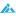# 如何设置根据指定概率获取范围内的随机数中奖概率推荐

08-26 275摘要： 假设：有一个二维数组，记录了所有本次抽奖的奖项信息： \$test_arr =array('a'=>20,'b'=>30,...

\$test_arr =array('a'=>20,'b'=>30,'c'=>50);

a奖概率20%，b奖概率30%，c奖概率50%

```private function get_rand(\$proArr) {
\$result = '';
//概率数组的总概率精度
\$proSum = array_sum(\$proArr);
//概率数组循环
foreach (\$proArr as \$key => \$proCur) {
\$randNum = mt_rand(1, \$proSum);
if (\$randNum <= \$proCur) {
\$result = \$key;
break;
} else {
\$proSum -= \$proCur;
}
}
unset (\$proArr);
return \$result;
}```

```/*
* 奖项数组
* 是一个二维数组，记录了所有本次抽奖的奖项信息，
* 其中id表示中奖等级，prize表示奖品，rate表示中奖概率。
* 注意其中的rate必须为整数，如果rate设置成0，即意味着该奖项抽中的几率是0，
* 数组中rate的总和（基数），基数越大越能体现概率的准确性。
* 本例中rate的总和为100，那么MAC对应的 中奖概率就是1%，
* 如果rate的总和是10000，那中奖概率就是万分之一了。
*/
\$prize_arr = array(
'0' => array('id'=>1,'prize'=>'MAC','rate'=>1),
'1' => array('id'=>2,'prize'=>'iPhone','rate'=>5),
'3' => array('id'=>4,'prize'=>'iWatch','rate'=>12),
'4' => array('id'=>5,'prize'=>'iPod','rate'=>22),
'5' => array('id'=>6,'prize'=>'抱歉!再接再厉','rate'=>50),
);

/*
* 每次前端页面的请求，PHP循环奖项设置数组，
* 通过概率计算函数get_rand获取抽中的奖项id。
* 将中奖奖品保存在数组\$res['yes']中，
* 而剩下的未中奖的信息保存在\$res['no']中。
*/
foreach (\$prize_arr as \$key => \$val) {
\$arr[\$val['id']] = \$val['rate'];
}
\$rid = get_rand(\$arr); //根据概率获取奖项id

\$res['yes'] = \$prize_arr[\$rid-1]['prize']; //中奖项
unset(\$prize_arr[\$rid-1]); //将中奖项从数组中剔除，剩下未中奖项
shuffle(\$prize_arr); //打乱数组顺序
for(\$i=0;\$i<count(\$prize_arr);\$i++){
\$pr[] = \$prize_arr[\$i]['prize'];
}
\$res['no'] = \$pr;   //未中奖项
print_r(\$res);```

### 觉得文章有用就打赏一下文章作者

#### 支付宝扫一扫打赏#### 微信扫一扫打赏#### 发表评论取消回复